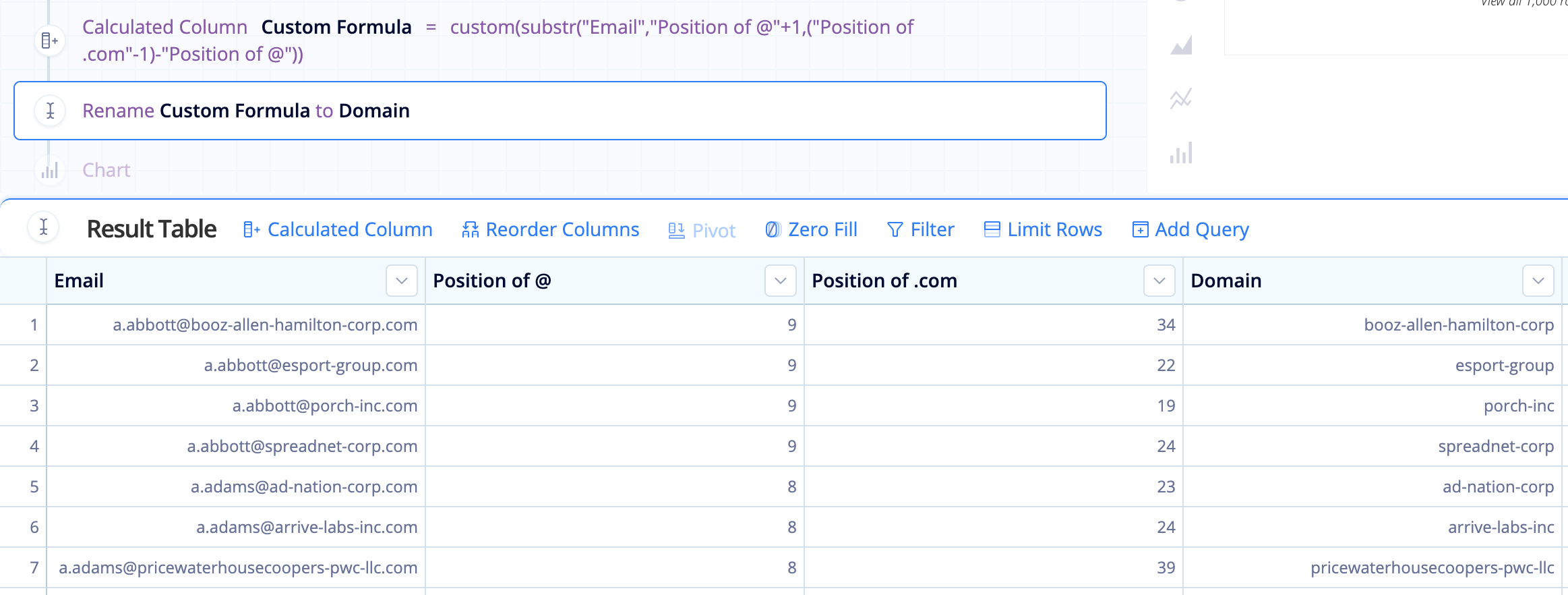# SQLite string functions in Visual SQL

SQLite is the most used database engine in the world and it powers Chartio’s Visual SQL Pipeline. There are a lot of core functions that can be used with string and numeric data types, but this covers some of the functions that work with strings (text).

## LENGTH(X)

``````length("Column Name")
``````

### When to use

When you need to know how many characters are in the string

### Example

In this example, we get the length of each Activity name:

``````length("Activity")
``````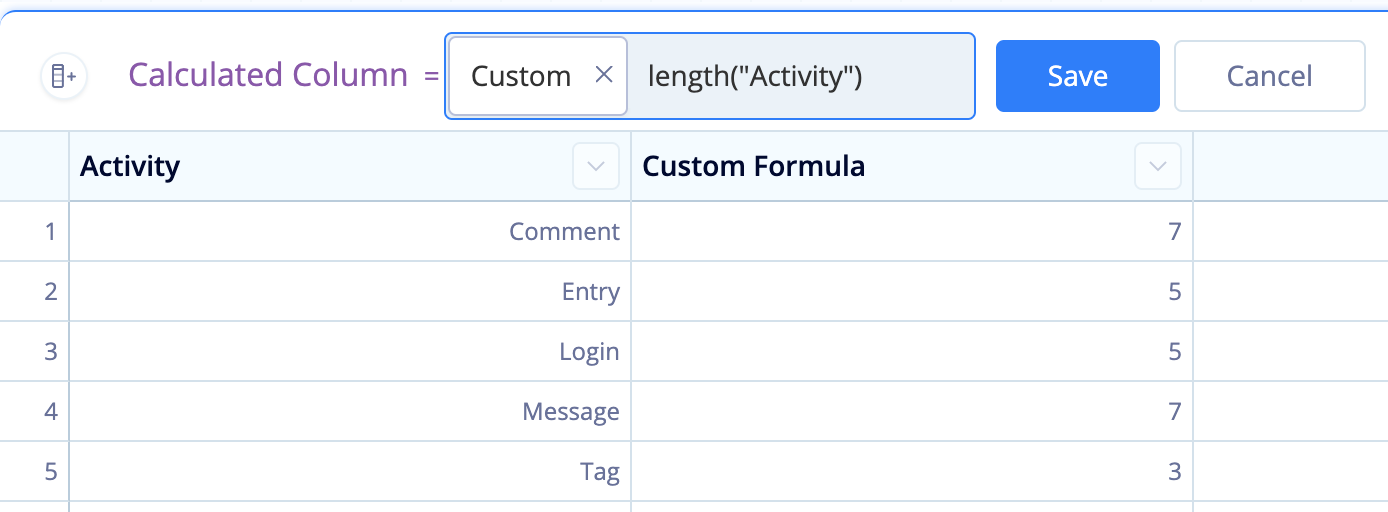## LOWER(X) / UPPER(X)

``````lower("Column Name")

upper("Column Name")
``````

### When to use

When you need to convert your string to all lowercase or all uppercase letters

### Example

In this example, we convert the city names to all lowercase letters:

``````lower("City")
``````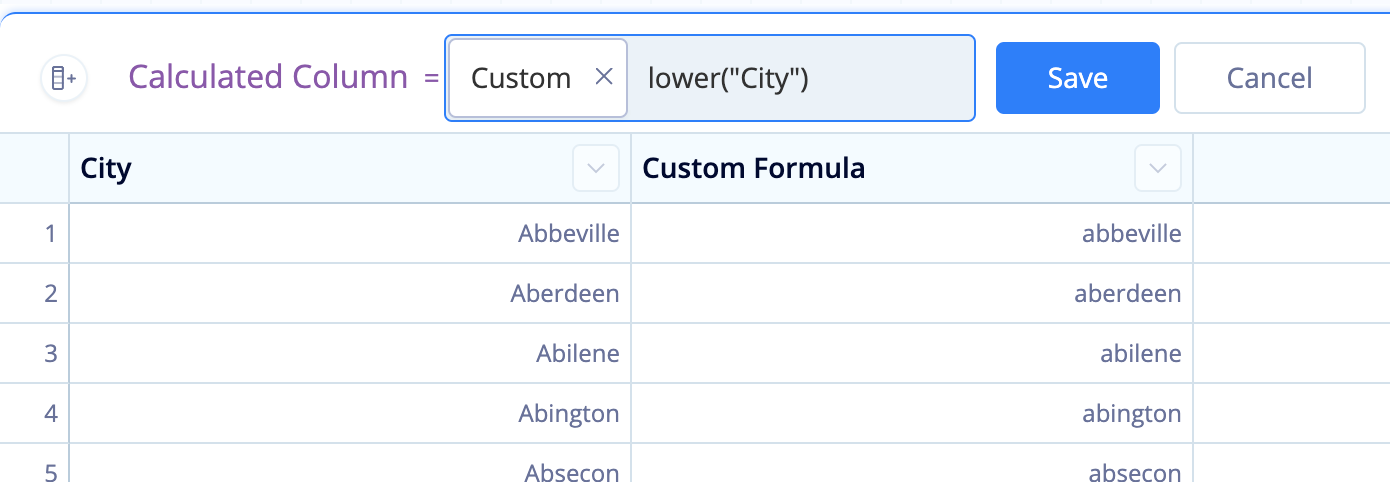## REPLACE(X, Y, Z)

``````replace("Column Name",'replace_this','with_this')
``````

### When to use

When you need to replace a character or substring in your string with another character or string

### How to use

• X represents the string that contains the character or string you want to replace.
• Y represents the character or substring you want to replace.
• Z represents the character or string that is used to replace Y.

### Example

In the example below, we are replacing the space in between the person’s first name and last name (`' '`) with dashes(`'-'`) in the Full Name column:

``````replace("Full Name",' ','-')
``````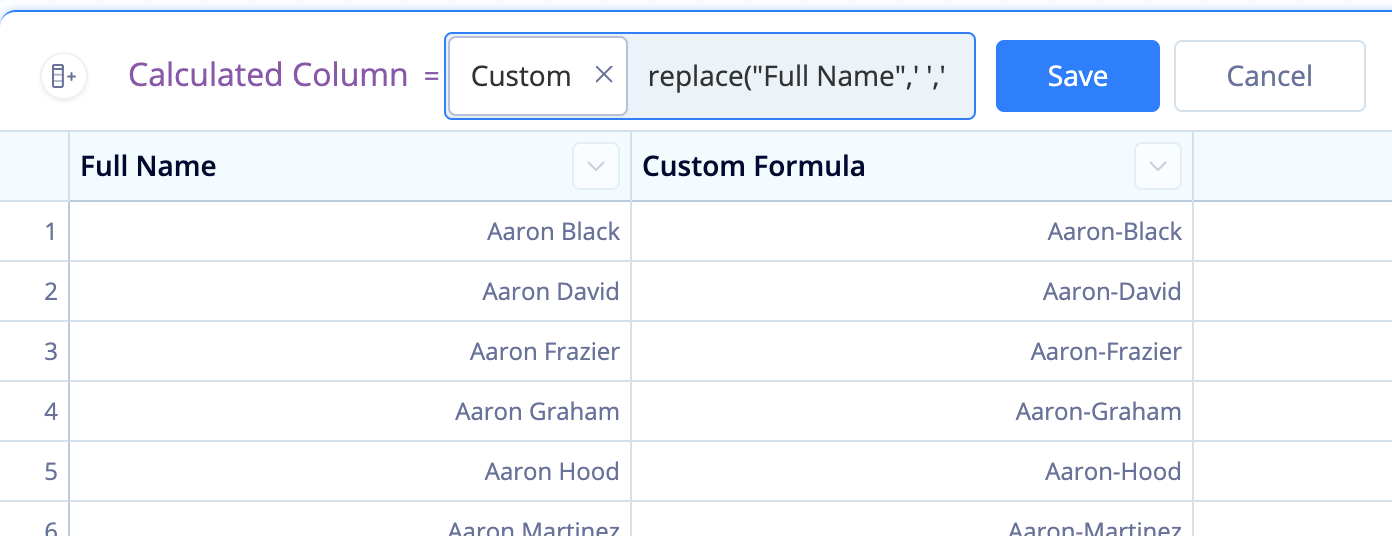## INSTR(X, Y)

``````instr("Column Name",'Character')
``````

### When to use

When you need to find the position of a character in a string

### How to use

• X represents the string that contains the character whose position you want to obtain.
• Y represents the character whose position you want to obtain.

Note: If the character appears multiple times in the string, the function returns the position of its first occurrence.

### Example

In this example, we want to know where the first space (`' '`) is located in the company’s name (Name column):

``````instr("Name",' ')
``````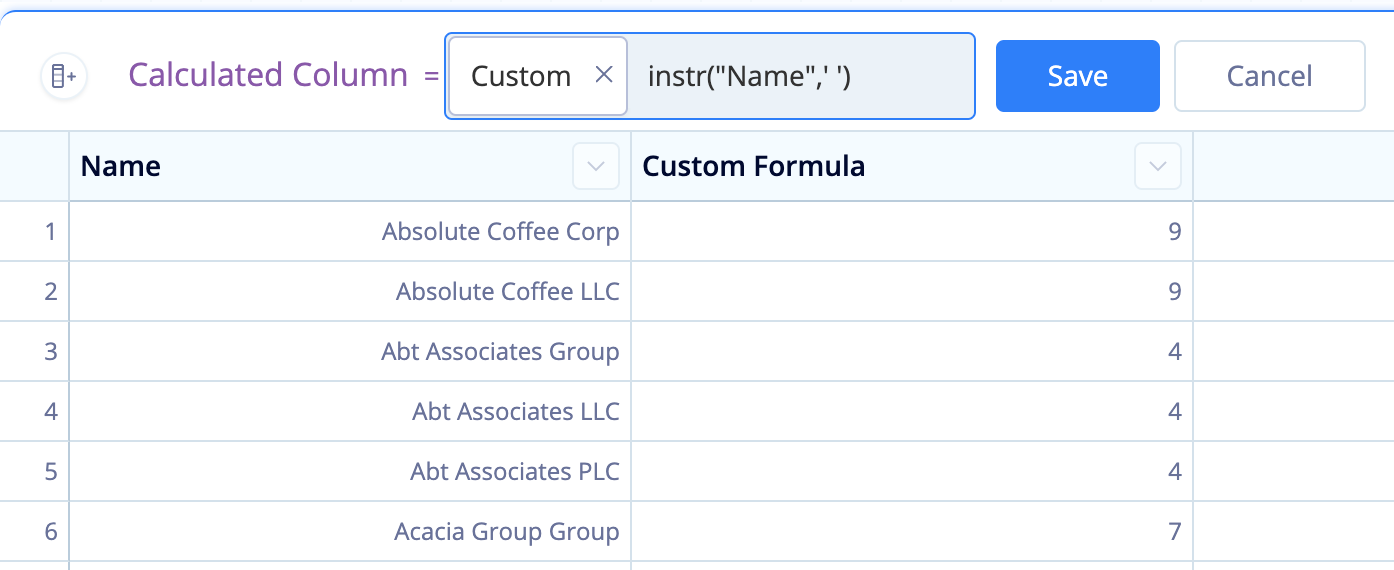## SUBSTR(X, Y, Z)

``````substr("Column Name",'start_position','end_position')
``````

### When to use

When you need to obtain a substring of the string you’re working with

### How to use

• X represents the string you want to obtain a substring from.
• Y represents the starting position to obtain the substring (the first character position in the string is always `1`).
• Z (optional; can be omitted) represents the number of characters in the resulting string.

### Example

If you have a phone number column in the format 5556667777, but you want the format (555) 666-7777, you can format it using `substr()`.

In Formula Column Action, select the Custom formula type and enter the following `substr()` function:

``````'('||substr("Phone",0,4)||')'||' '||substr("Phone",4,3)||'-'||substr("Phone",7,5)
``````

To customize it to your data, replace `Phone` with the name of the column you’re editing.

### Example 2

Here’s a more complicated example. Let’s say we want to pull the domain name from each user’s company email. We know emails follow this general format: `myname@mycompany.com`

To make the final `substr()` function a bit cleaner, let’s get two pieces of information using the `instr()` function:

• The position of the `@` in each email (We’ll name this column “Position of @”): instr(“Email”, ‘@’)
• The position of the `.com` in each email (We’ll name this column “Position of .com”): instr(“Email”, ‘.com’)

Now let’s figure out how to get the domain from the email.

• Our X value is “Email”—that one’s easy.
• Our Y value needs to be the position after the `@` position:

`````` "Position of @"+1
``````
• Our Z value needs to be the length of the domain, which we can get by subtracting our Y value from the position before the `.com` (i.e., `(Z-1)-Y`):

`````` ("Position of .com"-1)-"Position of @"
``````

This is our final substr() formula:

``````substr("Email","Position of @"+1,("Position of .com"-1)-"Position of @")
``````

Took a bit of effort, but we’re able to successfully get the domain names from each user’s email!